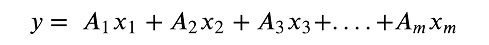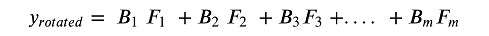# Finding the Great Predictors for Machine LearningFinding the Great Predictors for Machine Learning

Planning a data model takes a clear look at how variables should be used. A few techniques like factor analysis can help IT teams develop an efficient means to manage a model. Here’s how.Planning machine learning models often means you discover ways to refine the number of variables that inputs data to that model. Doing so reducing your analysis times. One choice you should consider for making your analysis efficient is a factor analysis. You right choice of a factor analysis can confirm if a model can be simplified.

Factor analysis is a statistical process for expressing variables in terms of latent variables called factors. Factors represent two or more variables that are highly correlated to each other. In short, factors are proxies for the model variables because of a common variance that exist because the variables correlate to each other.

The benefit of factor analysis is to eliminate variables that are not influencing the model. Factors developed when transforming the dimensionality of a dataset present a more economic way to describe influential variables.

The result is a reduced number of parameters for statistical models, be it a regression or a machine learning model. An analyst can plan a more optimal computation of training data, allowing a machine learning model to be developed more efficiently.

Factor analysis is particularly useful for surveys that contain a broad variety of comments and categorical responses. Survey responses are typically categorized, such as a Likert scale, in which respondents rate a question statement as 1 (very strongly agree) to 10 (very strongly disagree).  But interpreting which answers can influence a sought answer can be tricky to establish. Asking a battery of questions introduces complexity in determining what responses yield the strongest overall influence among survey respondents. Factor analysis can help develop the scoring into a statistical relationship that can indicate how to best rank responses from each question. Factor analysis is used extensively in psychology studies to understand attitudes and beliefs from surveys responses.

There are six assumptions that data must meet to develop a viable factor analysis model:

1. The observations appear as intervals. Nominal and ordinal observations do not work in a factor analysis.

2. The dataset must have an adequate structure. This means it contains at least 100 observations. There are also a high ratio of observations to variables, about twice as many observations as there are variables. The dataset should ensure that more variables than factors created.

3. No outliers exist in the dataset.

4. Variables are linear in nature.

5. No perfect multicollinearity exists, which means each variable is unique. Multicollinearity is essentially high intercorrelation among variables.

6. No homoscedasticity is needed between variables. Homoscedasticity means all variables have the same variance and, consequently, same size standard deviation.

Once you have checked your data against these guidelines, you can next work on your dataset to determine factors. You have a few selections for modeling tools depending on your programming proficiency. Libraries for R programming and Python are popular choices among data scientists and engineers. The arrangement offers flexibility in creating additional calculations and automating steps such as a querying updated data from a data lake. Another option is statistical software like SPSS. Statistical software contains pre-arranged settings to calculate factors, similar to basic statistical features in Excel.

In either case, you are transforming the columns into factors. So, if your variables are meant for a linear model; they may look like the following:where xm is the variable and Am is a coefficient to help relate one variable to another.

With the linear model in mind, factors are structured similarly with coefficients called factor loadings that provide the multiple for the factors in your models.To determine factor loading, your program or software will deploy a mathematical rotation. Rotations simplify how variables are examined to understand how many factors are possible.  Orthogonal rotation is a standard choice, usually indicating that two factors explaining the majority of variable variance. But orthogonal also emphasizes the first and second factors. Think of it as a having F1 and Fbut missing F3  that would increase accuracy and make the model truly optimal.

Thus, your actual work will require examining the data with various rotations types -- varimax, equimax, and oblimin, among others -- to judge the factor loadings that work best. Some rotation methods have specific correlation conditions. In those instances, packages from R and Python can apply the right rotation to your data.

The programs calculate eigenvalues, a scalar related to factor loadings. Eigenvalues measure the amount of variation for which a given factor accounts. It serves a purpose similar to that of a correlation coefficient among regression variables. A correlation coefficient expresses how related two given variables are. Factor loading demonstrates how related two factors are.

Your tools will arrange factors in decreasing or increasing order of eigenvalues.  Eigenvalues range from -1 to 1.  Eigenvalues greater than 0 means a factor explains more variance than the single variable. Eigenvalues close to zero implies multicollinearity, which you want to avoid for your model. Eigenvalues that are negative or zero reflect factors that can be potentially uninfluential.

The factor with the largest eigenvalue is the most influential, the second the second most, and so forth. With the factors identified you can remove the least influential and see how your model operates.

There are many kinds of factor analysis available. Exploratory factor analysis is a common choice for testing the number of factors without requiring a prior hypothesis on the variables. Yet a more complex technique, confirmatory factor analysis, tests the hypothesis that certain features in the dataset are associated with specific factors. In many instances you will find yourself comparing results from different rotation methodologies and data assumptions to see what factors best explains the variance of your variables and establishes the model.

The right data model will not land in your lap. You will need to learn what variables work and not work, dictating what data you will use for model. Ultimately, you will come closer to discovering your best model through factor analysis. You will discover the minimal variables necessary to make your model the right model for your needs.

Follow up with these articles on machine learning:

How to Keep Machine Learning Steady and Balanced

Pandemic Accelerates Machine Learning

Automating and Educating Business Processes with RPA, AI and ML